• 邻接表创建无向图

千次阅读 2019-05-31 17:39:16
1.首先要明白邻接表的存储结构 顶点集合（数组存储） 与顶点相连的其他顶点（链表存储） v0 -> v2->v3->v1 v1 ->v2->v0 v2 ->v0->v3->v1 v3 ->v0->...

1.首先要明白邻接表的存储结构

顶点集合（数组存储）           与顶点相连的其他顶点（链表存储）

v0    -> v2->v3->v1

v1    ->v2->v0

v2    ->v0->v3->v1

v3     ->v0->v2

2.顶点与边的数据结构

顶点的数据结构

public class VertexNode {
private String data;   //存放顶点结点信息   比如名称
private EdgeNode firstEdge;  //指向与该结点相连的 顶点集合 的第一个结点
//为了简洁，省略get,set方法
}

边的数据结构

public class EdgeNode {
private int weight;  //权值  对于非网图可以不需要  所谓网图：就是带权的图
private EdgeNode next; //下一个邻接点

//省略get,set方法

}

图的数据结构

private static final int MAX_SIZE = 100;        //能存放的最大顶点数
private VertexNode[] vertexNodes = new VertexNode[MAX_SIZE];   //存放顶点的数组
private int vertexNum;      //顶点数   不能超过能存放顶点数的最大值
private int edgeNum;          //边数   不能超过无向图的最大边数 即  n(n-1)/2   n为顶点数

//省略get,set方法

}

3.实现与测试

/**
* 测试：
*      创建一个无向图 顶点集（v0,v1,v2,v3,v4)  边集{(v0,v1),(v1,v2),(v2,v3),(v3,v0),(v0,v2)}
*      与顶点相关的其他结点插入的方法：头插法   比如  v0->v1   想插入v2 ,v3  1)v0->v2-v1 2)v0->v3->v2-v1
*      vo是边的起始点，也是每个链表的头结点
*/
public class GraphApplication {

public static void main(String[] args){
traverseGraph(g);
}

System.out.println("输入顶点数：");
Scanner sc = new Scanner(System.in);
int vertexNum = sc.nextInt();

System.out.println("输入边数（边数不能超过无向图的最大边数）：");
int edgeNum = sc.nextInt();
graph.setVertexNum(vertexNum);
graph.setEdgeNum(edgeNum);

//初始化顶点数组
for(int i = 0; i < vertexNum; i++){
VertexNode v = new VertexNode();
v.setData("v" + i);  //此处直接赋 v0  v1 ...值
graph.getVertexNodes()[i] = v;
}

//将各个顶点与边集进行连接
VertexNode[] vertexNodes = graph.getVertexNodes();
for(int i = 0; i < edgeNum; i++){
System.out.println("输入边（vi,vj）的起点");
int start = sc.nextInt();
System.out.println("输入边（vi,vj）的终点");
int end = sc.nextInt();

//以头插法的方式插入到链表中
EdgeNode edgeNodei = new EdgeNode();
edgeNodei.setNext(vertexNodes[start].getFirstEdge());
vertexNodes[start].setFirstEdge(edgeNodei);

//因为是无向图  vi到vj有边  那么 vj到vi也有边
EdgeNode edgeNodej = new EdgeNode();
edgeNodej.setNext(vertexNodes[end].getFirstEdge());
vertexNodes[end].setFirstEdge(edgeNodej);
}
return graph;
}

for(int i = 0; i < g.getVertexNodes().length; i++){
if(g.getVertexNodes()[i]  != null){
System.out.print("顶点" + g.getVertexNodes()[i].getData() + " : ");    //输出该顶点信息
EdgeNode node = g.getVertexNodes()[i].getFirstEdge();
while(node != null){
node = node.getNext();
}
System.out.println();
}
}
}

}

4.输出结果

按边的顺序进行输入(v0,v1),(v1,v2),(v2,v3),(v3,v0),(v0,v2)

顶点：v0 : v2-->v3-->v1-->
顶点：v1 : v2-->v0-->
顶点：v2 : v0-->v3-->v1-->
顶点：v3 : v0-->v2-->

突然发现，java的hash表好像就是用的邻接表，你们看是不是很像，顶点使用数组存储，与顶点相连的其他顶点用链表关联

展开全文邻接表创建无向图 无向图的创建 java实现
• 邻接表表示法： 邻接表(Adjacency List) 是的 一 种链式存储结构。在邻接表中，对图中每个顶点V建立一个单链表，把与 V相邻接的顶点放在这个链表中。邻接表中每个单链表的第一个结点存放有关顶点的信息， 把...

图的邻接表表示法：

邻接表(Adjacency List) 是图的 一 种链式存储结构。在邻接表中，对图中每个顶点V建立一个单链表，把与 V相邻接的顶点放在这个链表中。邻接表中每个单链表的第一个结点存放有关顶点的信息， 把这 一 结点看成链表的表头， 其余结点存放有关边的信息， 这样邻接表便由两部分组成：表头结点表和边表。

(1) 表头结点表：由所有表头结点以顺序结构的形式存储， 以便可以随机访问任 一 顶点的边链表。表头结点包括数据域 (data) 和链域 (firstarc) 两部分。其中， 数据域用于存储顶点 V 的名称或其他有关信息；链域用千指向链表中第 一 个结点（即与顶点 V邻接的第 一 个邻接点）。

(2)边表：由表示图中顶点间关系的 2n个边链表组成。边链表中边结点包括邻接点域

(adjvex) 、数据域 (info) 和链域 (nextarc) 三部分。其中， 邻接点域指示与顶点 V邻接的点在图中的位置；数据域存储和边相关的信息， 如权值等；链域指示与顶点v邻接的下 一 条边的结点。

 data firstarc

表头节点

边结点

【算法步骤】

1.输入总顶点数和总边数。

2.依次输入点的信息存入顶点表中，使每个表头结点的指针域初始化为NULL。

3.创建邻接表。依次输入每条边依附的两个顶点， 确定这两个顶点的序号i和j之后， 将此边结点分别插入 Vi 和vj 对应的两个边链表的头部。

//邻接矩阵法构造无向图
void CreateUGD(ALGraph &G){
printf("请输入图的总顶点数:");
cin>>G.vexnum;
printf("请输入图的总边数:");
cin>>G.arcnum;
//输入各点，构造表头结点表
for(int i=0;i<G.vexnum;i++){
printf("请输入第%d个顶点信息:",(i+1));
cin>>G.vertices[i].data;
G.vertices[i].firstarc=NULL;
}
//输入各边，构造邻接表
for(int k=0;k<G.arcnum;k++){
printf("输入第%d条边的信息:\n", (k+1));
printf("输入边依附的第一个顶点:");
VerTexType v1;
cin>>v1;
printf("输入边依附的第二个顶点:");
VerTexType v2;
cin>>v2;
//确定v1，v2在图G中的位置，即在G.vertices中的序号
int i= LocateVex(G, v1);
int j= LocateVex(G, v2);
struct ArcNode *p1, *p2;
p1=new ArcNode; //生成一个新的边结点
p1->nextarc=G.vertices[i].firstarc;
G.vertices[i].firstarc=p1;
p2=new ArcNode; //生成另一个对称边结点p2
p2->nextarc=G.vertices[j].firstarc;
G.vertices[j].firstarc=p2;
}
}

完整的实现代码如下：

#include<stdio.h>
#include<stack>
#include <iostream>
#define MAXSIZE 100
#define MaxInt 32767 //表示最大值，即正无穷大
#define MVNum 100 //定义最大顶点数
using namespace std;

typedef char VerTexType; //假设顶点数据类型为字符型
typedef int ArcType; //假设边的权值为整型
//定义边结点
typedef struct ArcNode{
struct ArcNode *nextarc; //下一条边的指针
}ArcNode;
//定义顶点结点信息
typedef struct VNode{
VerTexType data;
ArcNode *firstarc; //指向第一条依附该顶点的边的指针
//定义邻接表的结构
typedef struct {
int vexnum, arcnum; //当前图的点数和边数
}ALGraph;

//确定顶点vex在G.vertices中的序号
int LocateVex(ALGraph &G, VerTexType vex){
for(int i=0;i<G.vexnum;i++){
if(G.vertices[i].data==vex){
return i;
}
}
}

//邻接矩阵法构造无向图
void CreateUGD(ALGraph &G){
printf("请输入图的总顶点数:");
cin>>G.vexnum;
printf("请输入图的总边数:");
cin>>G.arcnum;
//输入各点，构造表头结点表
for(int i=0;i<G.vexnum;i++){
printf("请输入第%d个顶点信息:",(i+1));
cin>>G.vertices[i].data;
G.vertices[i].firstarc=NULL;
}
//输入各边，构造邻接表
for(int k=0;k<G.arcnum;k++){
printf("输入第%d条边的信息:\n", (k+1));
printf("输入边依附的第一个顶点:");
VerTexType v1;
cin>>v1;
printf("输入边依附的第二个顶点:");
VerTexType v2;
cin>>v2;
//确定v1，v2在图G中的位置，即在G.vertices中的序号
int i= LocateVex(G, v1);
int j= LocateVex(G, v2);
struct ArcNode *p1, *p2;
p1=new ArcNode; //生成一个新的边结点
p1->nextarc=G.vertices[i].firstarc;
G.vertices[i].firstarc=p1;
p2=new ArcNode; //生成另一个对称边结点p2
p2->nextarc=G.vertices[j].firstarc;
G.vertices[j].firstarc=p2;
}
}

//遍历图的邻接表
void PrintfG(ALGraph &G){
printf("遍历图的邻接表:\n");
for(int i=0;i<G.vexnum;i++){
printf("顶点%c ", G.vertices[i].data);
ArcNode *p;
p=G.vertices[i].firstarc;
while(p){
p=p->nextarc;
}
}
}

int main(){
ALGraph G;
CreateUGD(G);
PrintfG(G);
}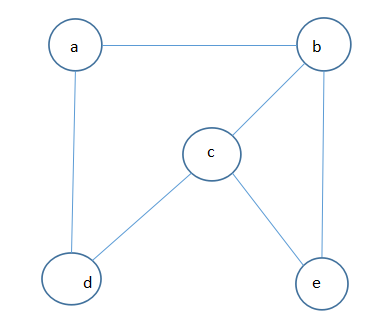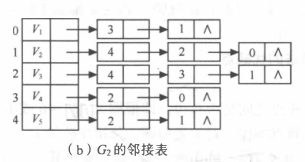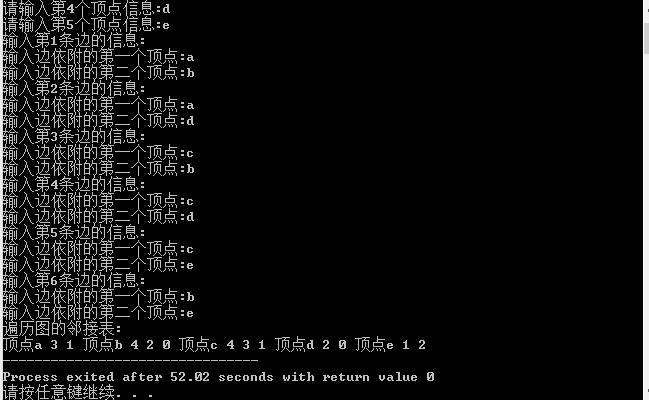邻接表法创建有向图的算法：

//邻接矩阵法构造有向图
void CreateUGD(ALGraph &G){
printf("请输入图的总顶点数:");
cin>>G.vexnum;
printf("请输入图的总边数:");
cin>>G.arcnum;
//输入各点，构造表头结点表
for(int i=0;i<G.vexnum;i++){
printf("请输入第%d个顶点信息:",(i+1));
cin>>G.vertices[i].data;
G.vertices[i].firstarc=NULL;
}
//输入各边，构造邻接表
for(int k=0;k<G.arcnum;k++){
printf("输入第%d条边的信息:\n", (k+1));
printf("输入边依附的第一个顶点:");
VerTexType v1;
cin>>v1;
printf("输入边依附的第二个顶点:");
VerTexType v2;
cin>>v2;
//确定v1，v2在图G中的位置，即在G.vertices中的序号
int i= LocateVex(G, v1);
int j= LocateVex(G, v2);
struct ArcNode *p1, *p2;
p1=new ArcNode; //生成一个新的边结点
p1->nextarc=G.vertices[i].firstarc;
G.vertices[i].firstarc=p1;
//与无向图相反，有向图不需要生成对称边结点
//p2=new ArcNode; //生成另一个对称边结点p2
//p2->nextarc=G.vertices[j].firstarc;
//G.vertices[j].firstarc=p2;
}
}

完整的代码实现如下：

#include<stdio.h>
#include<stack>
#include <iostream>
#define MAXSIZE 100
#define MaxInt 32767 //表示最大值，即正无穷大
#define MVNum 100 //定义最大顶点数
using namespace std;

typedef char VerTexType; //假设顶点数据类型为字符型
typedef int ArcType; //假设边的权值为整型
//定义边结点
typedef struct ArcNode{
struct ArcNode *nextarc; //下一条边的指针
}ArcNode;
//定义顶点结点信息
typedef struct VNode{
VerTexType data;
ArcNode *firstarc; //指向第一条依附该顶点的边的指针
//定义邻接表的结构
typedef struct {
int vexnum, arcnum; //当前图的点数和边数
}ALGraph;

//确定顶点vex在G.vertices中的序号
int LocateVex(ALGraph &G, VerTexType vex){
for(int i=0;i<G.vexnum;i++){
if(G.vertices[i].data==vex){
return i;
}
}
}

//邻接矩阵法构造有向图
void CreateUGD(ALGraph &G){
printf("请输入图的总顶点数:");
cin>>G.vexnum;
printf("请输入图的总边数:");
cin>>G.arcnum;
//输入各点，构造表头结点表
for(int i=0;i<G.vexnum;i++){
printf("请输入第%d个顶点信息:",(i+1));
cin>>G.vertices[i].data;
G.vertices[i].firstarc=NULL;
}
//输入各边，构造邻接表
for(int k=0;k<G.arcnum;k++){
printf("输入第%d条边的信息:\n", (k+1));
printf("输入边依附的第一个顶点:");
VerTexType v1;
cin>>v1;
printf("输入边依附的第二个顶点:");
VerTexType v2;
cin>>v2;
//确定v1，v2在图G中的位置，即在G.vertices中的序号
int i= LocateVex(G, v1);
int j= LocateVex(G, v2);
struct ArcNode *p1, *p2;
p1=new ArcNode; //生成一个新的边结点
p1->nextarc=G.vertices[i].firstarc;
G.vertices[i].firstarc=p1;
//p2=new ArcNode; //生成另一个对称边结点p2
//p2->nextarc=G.vertices[j].firstarc;
//G.vertices[j].firstarc=p2;
}
}

//遍历图的邻接表
void PrintfG(ALGraph &G){
printf("遍历图的邻接表:\n");
for(int i=0;i<G.vexnum;i++){
printf("顶点%c ", G.vertices[i].data);
ArcNode *p;
p=G.vertices[i].firstarc;
while(p){
p=p->nextarc;
}
}
}

int main(){
ALGraph G;
CreateUGD(G);
PrintfG(G);
}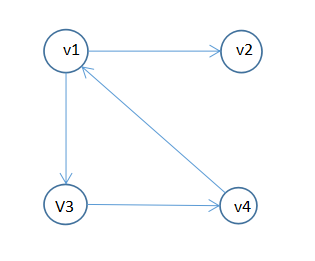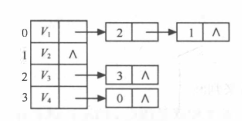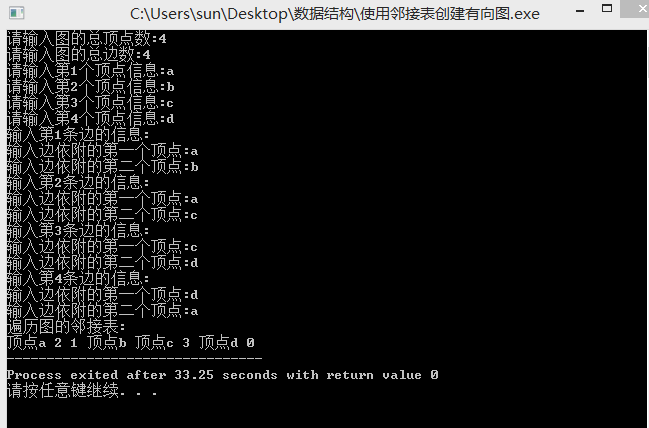展开全文• 7-2 邻接表创建无向图 (20 分)

千次阅读 2019-11-10 19:57:18
采用邻接表创建无向图G ，依次输出各顶点的度。 输入格式: 输入第一行中给出2个整数i(0<i≤10)，j(j≥0)，分别为图G的顶点数和边数。 输入第二行为顶点的信息，每个顶点只能用一个字符表示。 依次输入j行，每行...

采用邻接表创建无向图G ，依次输出各顶点的度。
输入格式:

输入第一行中给出2个整数i(0<i≤10)，j(j≥0)，分别为图G的顶点数和边数。 输入第二行为顶点的信息，每个顶点只能用一个字符表示。 依次输入j行，每行输入一条边依附的顶点。
输出格式:

依次输出各顶点的度，行末没有最后的空格。
输入样例:

5 7
ABCDE
AB
BC
BE
CD
CE
DE
输出样例:

2 3 3 3 3

#include<bits/stdc++.h>
using namespace std;

typedef struct ENode{/表结点
char data;
struct ENode *next;
}ENode;

typedef struct VNode{  //头结点
char data;
ENode *fistedges;
}VNode,vertex;

typedef struct{
vertex v;
int numNode,numedges;
}  Graph;

int find(Graph G,int c) 找弧尾
{
for(int i=0;i<G.numNode;i++)
{
if(G.v[i].data==c)
return i;
}
return -1;
}

void Create(Graph G)
{
cin>>G.numNode>>G.numedges;

for(int i=0;i<G.numNode;i++)保存顶点
{
char a;
cin>>a;
G.v[i].data=a;
G.v[i].fistedges=NULL;
}

for(int i=0;i<G.numedges;i++)/保存边
{
char a,b;
cin>>a>>b;
int p=find(G,a);
int q=find(G,b);
ENode *pre=(ENode*)malloc(sizeof(ENode));

/将b接在表头a的后面///
pre=G.v[p].fistedges;
if(pre==NULL)
{
ENode *qre=(ENode*)malloc(sizeof(ENode));
qre->data=b;
qre->next=NULL;
G.v[p].fistedges=qre;
}
else
{
while(pre->next!=NULL)
{
pre=pre->next;
}
ENode *qre=(ENode*)malloc(sizeof(ENode));
qre->data=b;
qre->next=NULL;
pre->next=qre;
}
//将a接到表头b的后面///
pre=G.v[q].fistedges;
if(pre==NULL)
{
ENode *qre=(ENode*)malloc(sizeof(ENode));
qre->data=a;
qre->next=NULL;
G.v[q].fistedges=qre;
}
else
{
while(pre->next!=NULL)
{
pre=pre->next;
}
ENode *qre=(ENode*)malloc(sizeof(ENode));
qre->data=a;
qre->next=NULL;
pre->next=qre;
}
}
///输出各个顶点的度///
for(int i=0;i<G.numNode;i++)
{
int s=0;
for(ENode *p=G.v[i].fistedges;p!=NULL;p=p->next)
{
s++;
}
if(i==0)
cout<<s;
else
cout<<" "<<s;
}
}

int main()
{
Graph G;
Create(G);
return 0;
}

展开全文• PTA 7-2 邻接表创建无向图 (20分) 采用邻接表创建无向图G ，依次输出各顶点的度。 输入格式: 输入第一行中给出2个整数i(0<i≤10)，j(j≥0)，分别为图G的顶点数和边数。 输入第二行为顶点的信息，每个顶点只能用一...

PTA 7-2 邻接表创建无向图 (20分)

采用邻接表创建无向图G ，依次输出各顶点的度。

输入格式:

输入第一行中给出2个整数i(0<i≤10)，j(j≥0)，分别为图G的顶点数和边数。 输入第二行为顶点的信息，每个顶点只能用一个字符表示。 依次输入j行，每行输入一条边依附的顶点。

输出格式:

依次输出各顶点的度，行末没有最后的空格。

5 7
ABCDE
AB
BC
BE
CD
CE
DE

2 3 3 3 3

无需建图

#include<bits/stdc++.h>
using namespace std;
int main(){
map<char, int> m;
int i, j;
char G, a, b;
scanf("%d %d",&i,&j);
getchar();
scanf("%s",G);
getchar();
while(j--) {
scanf("%c%c",&a,&b);
getchar();
m[a]++;
m[b]++;
}
cout<<m[G];
for(int k = 1; k < i; k++)
cout<<' '<<m[G[k]];
return 0;
}

建图

#include<bits/stdc++.h>
using namespace std;
struct Graph {
char data;
struct Graph *next;
};
int i, j;
int search(struct Graph p) {
int c = 0;
c++;
}
return c;
}
int main(){
map<char, int> m;
char  a, b;
struct Graph ls;
scanf("%d %d",&i,&j);
getchar();
for(int k = 0; k <i; k++) {
cin>>a;
m[a] = k;
ls[k].next = NULL;
}
getchar();
while(j--) {
scanf("%c%c",&a,&b);
getchar();
struct Graph *t = new struct Graph;
t->data = b;
t->next = ls[m[a]].next;
ls[m[a]].next = t;
t = new struct Graph;
t->data = a;
t->next = ls[m[b]].next;
ls[m[b]].next = t;
}
cout<<search(ls);
for(int k = 1; k < i; k++)
cout<<' '<<search(ls[k]);
return 0;
}

展开全文c语言 c++
• 7-4 邻接表创建无向图 (20分)

千次阅读 2019-12-10 17:01:17
7-4 邻接表创建无向图 (20分) 采用邻接表创建无向图G ，依次输出各顶点的度。 输入格式: 输入第一行中给出2个整数i(0<i≤10)，j(j≥0)，分别为图G的顶点数和边数。 输入第二行为顶点的信息，每个顶点只能用一个...C++
• 7-1 邻接表创建无向图 (20分)

千次阅读 2020-02-23 11:53:43
7-1 邻接表创建无向图 (20分) 输入格式: 输入第一行中给出2个整数i(0<i≤10)，j(j≥0)，分别为图G的顶点数和边数。 输入第二行为顶点的信息，每个顶点只能用一个字符表示。 依次输入j行，每行输入一条边依附的...
• #include<stdio.h> #include<iostream.h> #define MaxInt 32767 #define MVNum 100 #define OK 1 typedef char VerTexType;...typedef struct ArcNode//边 { int adjvex; ...
• 采用邻接表创建无向图G ，依次输出各顶点的度。 其中 G 是采用邻接表表示的无向图。 函数定义接口： void CreateUDG(ALGraph &G); 输入格式： 输入第一行中给出2个整数i(0<i≤10)，j(j≥0)，分别为图G的...c++ 数据结构
• 6-2 采用邻接表创建无向图 (20分) 采用邻接表创建无向图G ，依次输出各顶点的度。 函数接口定义： void CreateUDG(ALGraph &G); 其中 G 是采用邻接表表示的无向图。 裁判测试程序样例： #include <stdio.h&...数据结构
• 采用邻接表创建无向图G ，依次输出各顶点的度。 输入格式: 输入第一行中给出2个整数i(0<i≤10)，j(j≥0)，分别为图G的顶点数和边数。 输入第二行为顶点的信息，每个顶点只能用一个字符表示。 依次输入j行，每行...数据结构 算法
• 7-1 邻接表创建无向图 (20 分)

千次阅读 2019-10-24 17:15:55
采用邻接表创建无向图G ，依次输出各顶点的度。 输入格式: 输入第一行中给出2个整数i(0<i≤10)，j(j≥0)，分别为图G的顶点数和边数。 输入第二行为顶点的信息，每个顶点只能用一个字符表示。 依次输入j行，每行...C
• 主要介绍了邻接表无向图的Java语言实现完整源码，具有一定借鉴价值，需要的朋友可以参考下。
• 文章目录邻接矩阵创建无向网代码实现邻接表创建无向图代码实现 邻接矩阵创建无向网代码实现 #include<iostream> using namespace std; #define OK 1 #define Maxint 32767 typedef int status ; typedef ...数据结构
• //1图的邻接矩阵表示法和邻接表创建无向图存储 #include"pch.h" #include<iostream> using namespace std; #define MaxNum 100 //最大顶点数 //1.1图的邻接矩阵表示法创建无向图存储 #define MaxInt 32767 /...
• #include <iostream> #include <stdlib.h> using namespace std; ...//指向该顶点的下一个邻接点 }EdgeNode; typedef struct VertexNode//顶点结点 { char data;//顶点 EdgeN...深度优先 链表 数据结构
• 采用邻接表表示法创建无向图

千次阅读 多人点赞 2020-08-08 16:16:33
//采用邻接表表示法创建无向图 #include <iostream> using namespace std; #define MVNum 100 //最大顶点数 #define OK 1 typedef char VerTexType; //-==顶点信息 typedef int OtherInfo; //...指针 链表 c++
• 利用邻接表创建无向图

千次阅读 2021-12-15 21:47:00
} int main() { cout 邻接表创建无向图" ; ALGraph G; CreateUDG(G); for (int i = 0; i ; i++) { VNode temp = G.vertices[i]; ArcNode* p = temp.firststarc; if (!p) { cout [i].data ; } else { cout ; while ...c++ 数据结构
• 关于内部类的使用，使邻接表的结构定义更加清晰，不分散。在同一个类中实例化内部类和普通类的实例化相同，但在其他类中应用，实例化时需要加上外部实例化对象。例： OutClassout=newOutClass(); OutClass....java 数据结构
• 本文我们用C 邻接表实现无向图。用向量来存储一条邻接链表，存储可连通值。实现了判断是否连通，添加边，添加顶点的功能。UnDirectGraph.h#pragma onceinclude “stdafx.h”include using namespace st...
• C语言邻接表表示法创建无向图并输出 邻接表是图的一种链式存储结构，对图的每个顶点建立一个单链表，单链表第一个结点存放顶点信息，其余存放有关边信息。 邻接表由表头结点表和边表组成。 邻接表存储结构 #include ...数据结构 c语言 链表
• 邻接表来表示要创造的结构体太多了，我感觉其实有更简单的结构体来表示。 #include<stdio.h> #include <stdlib.h> #include <string.h> #include<malloc.h> //创建邻接表 //这个边的...
• 邻接表创建无向图（C++实现） #include <stdio.h> #include <iostream> using namespace std; #define MAXSIZE 100 //最大顶点数 typedef struct ArcNode{ //边节点信息 int adjvex; //该边所指向.....指针 链表 数据结构 单链表
• 邻接表法创建无向图（C语言）

万次阅读 多人点赞 2020-06-09 13:24:18
本题要求建立一个无向图，采用邻接表做为存储结构。 例如： 输入信息为：第一行给出图的顶点数n和边数e。第二行给出n个字符，表示n个顶点的数据元素的值。后面是e行，给出每一条边的两个顶点编号。 输出每个顶点的...数据结构 c语言
• 主要介绍了Python根据已知邻接矩阵绘制无向图操作,涉及Python使用networkx、matplotlib进行数值运算与图形绘制相关操作技巧,需要的朋友可以参考下
• 邻接表存储结构建立无向图》由会员分享，可在线阅读，更多相关《邻接表存储结构建立无向图(3页珍藏版)》请在人人文库网上搜索。1、算法功能：采用邻接表存储结构建立无向图 #include #include #define OK 1#define...数据结构
• 本章介绍邻接表无向图。在"图的理论基础"中已经对图进行了理论介绍，这里就不再对图的概念进行重复说明了。和以往一样，本文会先给出C语言的实现；后续再分别给出C++和Java版本的实现。实现的语言虽不同，但是原理...c语言邻接表的构建...